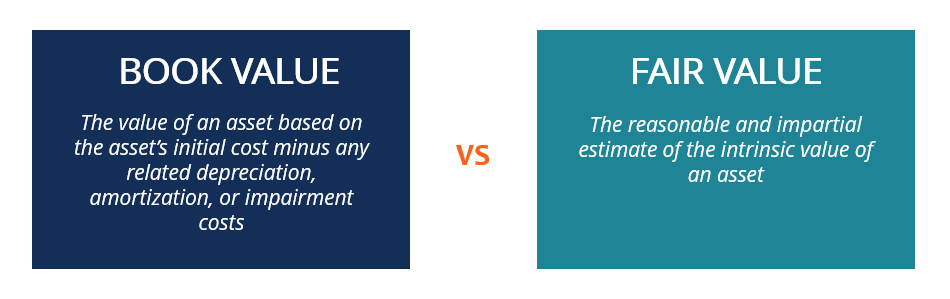# Book Value vs Fair Value

Learn about the differences between the two concepts

# What is Book Value vs Fair Value?

In accounting and finance, it is important to understand the differences between book value vs fair value. Both concepts are used in the valuation of an asset, but they refer to different aspects of an asset’s value. In this article, we will discuss book value vs fair value in detail and indicate their key distinctions.Book value indicates an asset’s value that is recognized on the balance sheet. Essentially, book value is the original cost of an asset minus any depreciation, amortization, or impairment costs.

On the other hand, fair value is referred to as an estimate of the potential value of an asset. In other words, it is the intrinsic value of an asset.

### What is Book Value?

Book value (also known as carrying value or net asset value) is the value of an asset that is recognized on the balance sheet. It is determined as the cost paid for acquiring an asset minus any depreciation, amortization, or impairment costs applicable to the asset. The concept of book value arises from the practice of recording the assets on the balance sheet at its historical cost.

Book value is one of the most important concepts in accounting. Firstly, book value determines the value of an asset recorded on a company’s balance sheet. Since stockholders’ equity is calculated as the difference between the assets’ and liabilities’ values, the book value is used to determine the equity value attributable to the company’s shareholders.

Note that the book value of assets indicates the value that shareholders would receive in case of the company’s liquidation. In addition, the book value is commonly utilized to demonstrate whether an asset is over- or underpriced. It is usually done by finding the difference between the asset’s book and market values.

### What is Fair Value?

Fair value is a reasonable and unbiased estimate of the intrinsic value of an asset. Essentially, the fair value of an asset is based on several factors such as utility, related costs, and supply and demand considerations. Another common definition of fair value is the price that would be obtained for the sale of an asset or paid to transfer a liability in a transaction between the market participants at the measurement date.

Essentially, the estimation of an asset’s fair value is a generally complicated process. Determining the asset’s fair value is generally guided by the accounting standards. IFRS and US GAAP provide guidance on how to measure the fair value of an asset.

Note that in accounting, the concept of the fair value is not applicable to all assets. Fair value is usually estimated for assets that are not recorded at their book value. Accounting using fair values is frequently exposed to potential accounting fraud due to the fact that companies can manipulate the fair value calculations.

CFI offers the Financial Modeling & Valuation Analyst (FMVA)™ certification program for those looking to take their careers to the next level. To keep learning and advancing your career, the following resources will be helpful:

• Depreciation Methods
• Enterprise Value vs Equity Value
• Modified Book Value
• Top Accounting Scandals